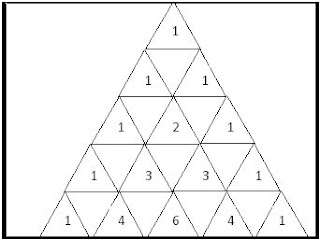## Saturday, May 23, 2009

### PASCAL TRIANGLE

Pascal's triangle is a geometric arrangement of the binomial cofficients in a triangle.The triangle is bordered by ones on the right and left sides, and each interior entry is the sum of the two entries above.Construction:-
At the top of Pascal's Triangle it have number 1 which is the zeroth row. Add the number directly above and to the left with the number directly above and to the right to find the new value. If either the number to the right or left is not present, substitute a zero in its place.
First Row 0+1=1,1+0=1
Second Row 0+1=1,1+1=2,1+0=1
Third Row 0+1=1,1+2=3,2+1=3,1+0=1
And so on the rows go infinitely.
Some properties:-
Sums of the numbers in Row
The sum of the numbers in any row is equal to nth power of 2, when n is the number of the row.
For example:and so on
Prime Numbers:-

The 0th element of every row is 1 and if the first element in any row is prime, then all the numbers in that row excluding 1’s are divisible by it .
For example:
In row5( 1 5 10 10 5 1)5,10 are divisible by 5.

If a diagonal of numbers of any length is selected starting at any of the 1's bordering the sides of the triangle and ending on any number inside the triangle on that diagonal, the sum of the numbers inside the selection is equal to the number below the end of the selection that is not on the same diagonal itself.
For example:1+6+21+56 = 84
1+7+28+84+210+462+924 = 1716
1+12 = 13
Powers of 11’s:-